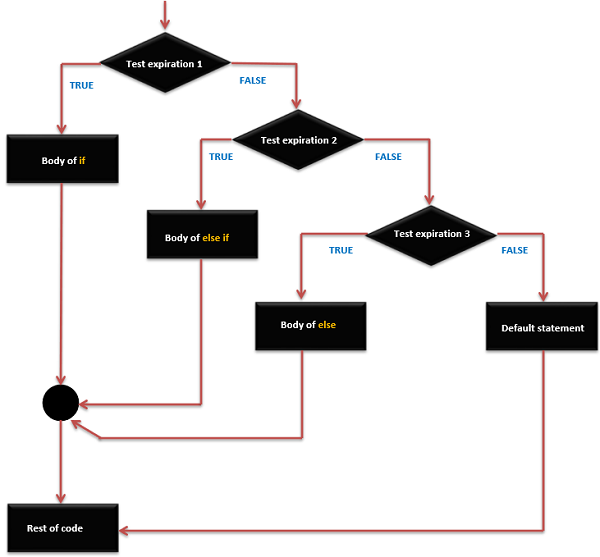# Arduino - If…else if …else statement

The if statement can be followed by an optional else if...else statement, which is very useful to test various conditions using single if...else if statement.

When using if...else if…else statements, keep in mind −

• An if can have zero or one else statement and it must come after any else if's.

• An if can have zero to many else if statements and they must come before the else.

• Once an else if succeeds, none of the remaining else if or else statements will be tested.

## if … else if …else Statements Syntax

```if (expression_1) {
Block of statements;
}

else if(expression_2) {
Block of statements;
}
.
.
.

else {
Block of statements;
}
```

## if … else if … else Statement Execution Sequence### Example

```/* Global variable definition */
int A = 5 ;
int B = 9 ;
int c = 15;

Void setup () {

}

Void loop () {
/* check the boolean condition */
if (A > B) /* if condition is true then execute the following statement*/ {
A++;
}
/* check the boolean condition */
else if ((A == B )||( B < c) ) /* if condition is true then
execute the following statement*/ {
C = B* A;
}else
c++;
}
```
arduino_control_statements.htm